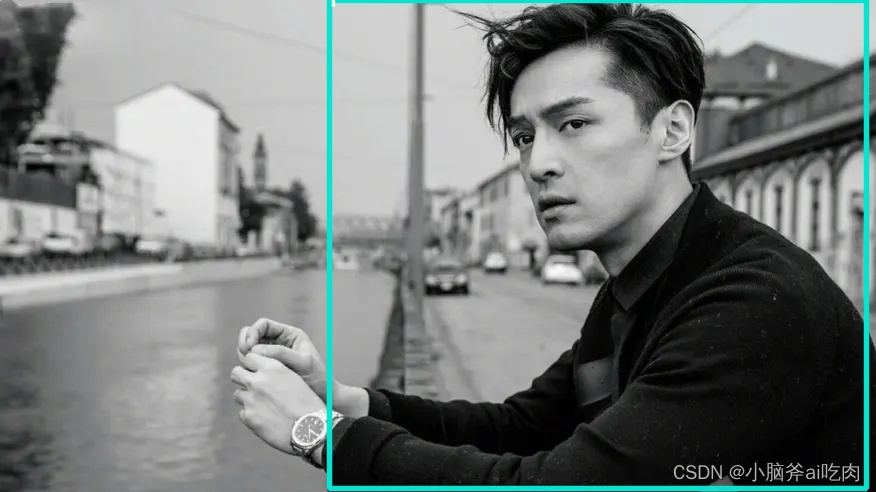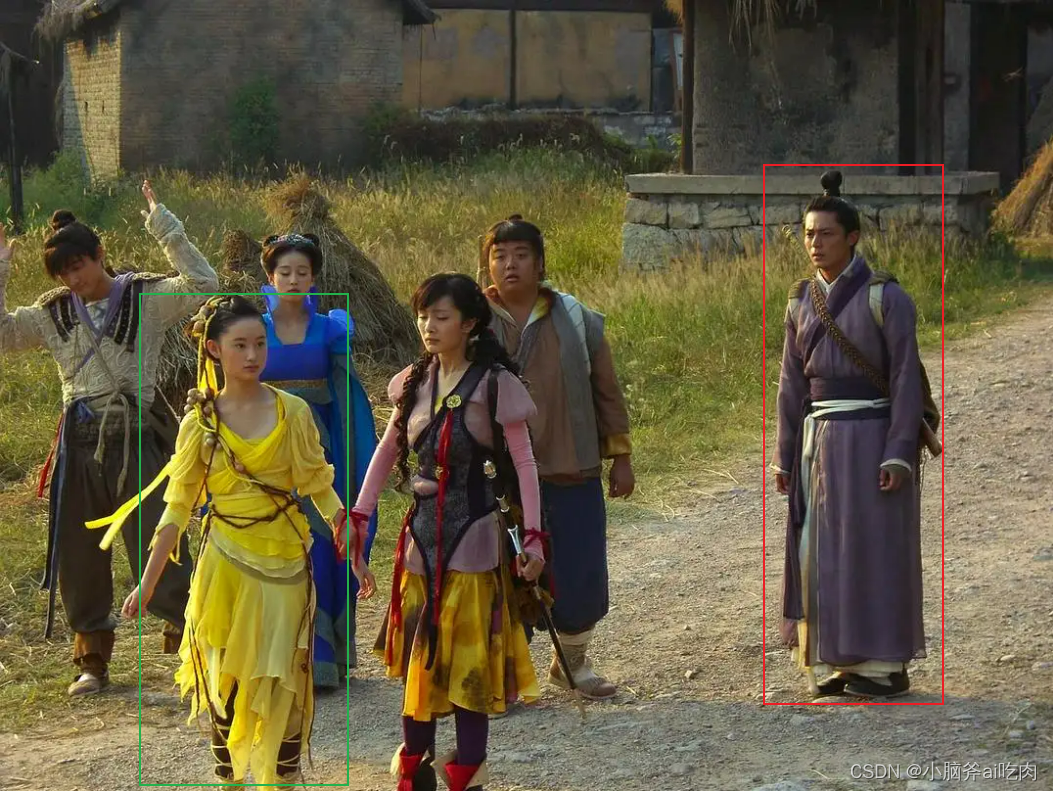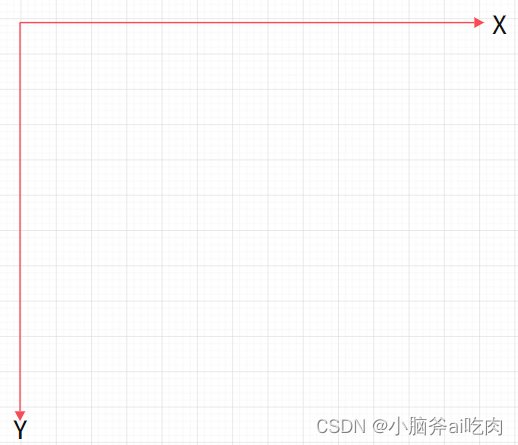# 利用yolov5进行目标检测，并将检测到的目标裁剪出来

## 利用yolov5进行目标检测，并将检测到的目标裁剪出来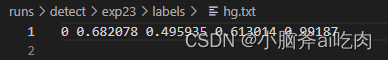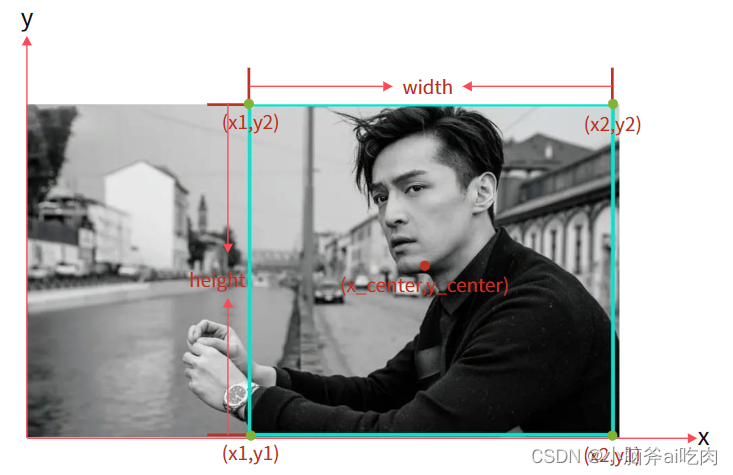``````x1=x_center-width/2
x2=x_center+width/2
y1=y_center-height/2
y2=y_center+height/2
``````

``````x1=（x_center-width/2）*整张图片的宽度(注：是整张图片的宽度不是目标的宽度,这里的宽度就是像素宽度)
x2=(x_center+width/2)*整张图片的宽度
y1=(y_center-height/2)*整张图片的高度（注：是整张图片的高度不是目标的高度,这里的高度就是像素高度）
y2=(y_center+height/2)*整张图片的高度
``````

``````import os
import cv2

def main():
#图片路径
img_path = './data/images/hg.jpg'
#txt文件路径
label_path = './runs/detect/exp23/labels/hg.txt'
# 读取图片，结果为三维数组
# 图片宽度(像素)
w = img.shape
# 图片高度(像素)
h = img.shape
# 打开文件，编码格式'utf-8','r+'读写
f = open(label_path, 'r+', encoding='utf-8')
# 读取txt文件中的第一行,数据类型str
# 根据空格切割字符串，最后得到的是一个list
msg = line.split(" ")
x1 = int((float(msg) - float(msg) / 2) * w)  # x_center - width/2
y1 = int((float(msg) - float(msg) / 2) * h)  # y_center - height/2
x2 = int((float(msg) + float(msg) / 2) * w)  # x_center + width/2
y2 = int((float(msg) + float(msg) / 2) * h)  # y_center + height/2
print(x1, ",", y1, ",", x2, ",", y2)
#裁剪
img_roi = img[y1:y2,x1:x2]
save_path='./cutpictures/hg.jpg'
cv2.imwrite(save_path,img_roi)

if __name__ == '__main__':
main()

``````